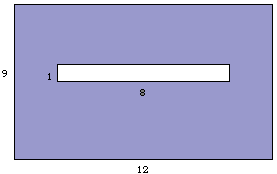# Cut Twice To Make a SquareTake a 12x9 rectangle and cut an 8x1 hole in the middle, with the hole centered exactly in the rectangle (I think of 12 and 8 as being the horizontal dimensions). Can you make two cuts so that the resulting pieces can be rearranged to form a square?

The knife used to make the cuts is not restricted to cutting along a straight line: it can turn as it cuts.

The Mathematica code for the diagram is attached below.

Source: G. Polya: Mathematical Discovery

```Show[Graphics[{GrayLevel[0.5],
Rectangle[{0, 0}, {12, 9}],
GrayLevel, Rectangle[{2, 4.5}, {10, 5.5}],
GrayLevel,
Line[{{0, 0}, {12, 0}, {12, 9}, {0, 9}, {0, 0}}],
Line[{{2, 4.5}, {10, 4.5}, {10, 5.5},
{2, 5.5}, {2,4.5}}],
Text[12, {6, -0.6}],
Text[9, {-0.4, 5}],
Text[1, {1.7, 4.8}],
Text[8, {6, 3.9}]}],
TextStyle -> {FontSize -> 12,
FontWeight -> Bold, FontFamily -> "Times"}];
```